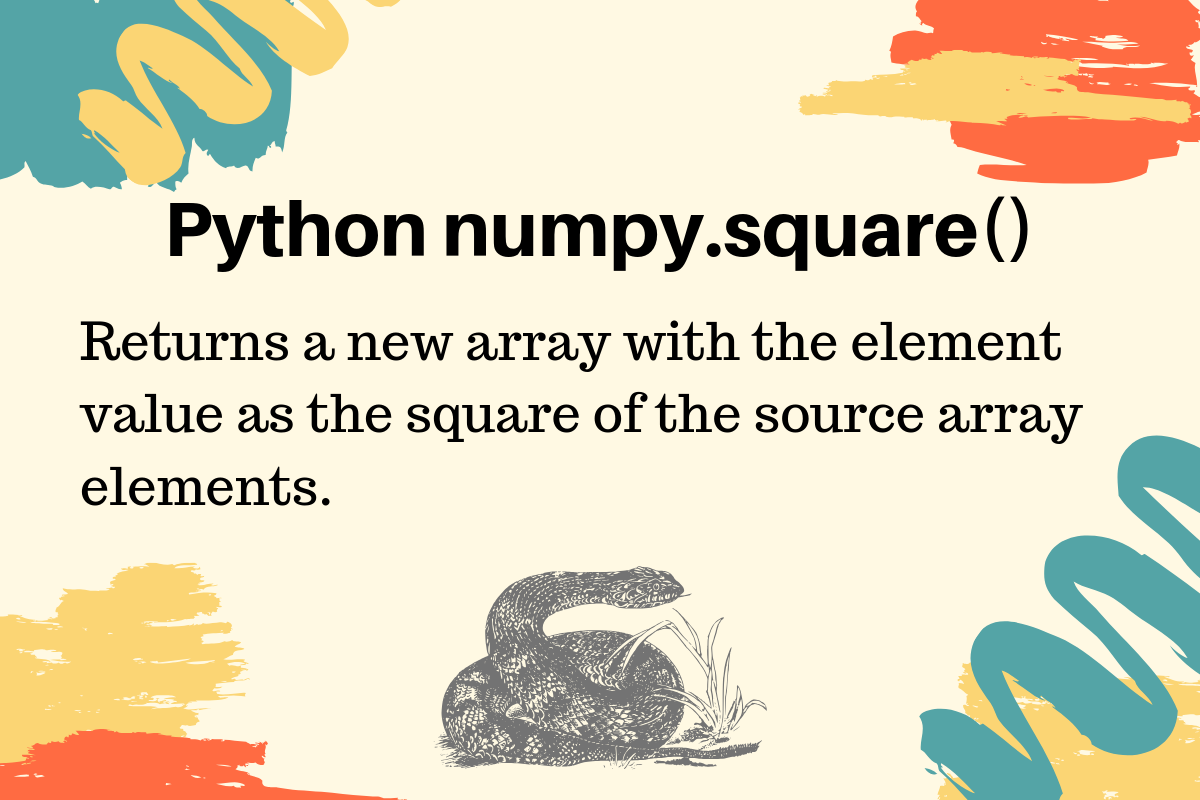# numpy.square() in Python

Published on August 3, 2022By PankajWhile we believe that this content benefits our community, we have not yet thoroughly reviewed it. If you have any suggestions for improvements, please let us know by clicking the “report an issue“ button at the bottom of the tutorial.

Python numpy.square() function returns a new array with the element value as the square of the source array elements. The source array remains unchanged.

## Python numpy.square() Examples

It’s a utility function to quickly get the square of the matrix elements. Let’s look at the examples of numpy square() function with integer, float, and complex type array elements.

### 1. numpy square() int array

``````import numpy as np

# ints
array_2d = np.array([[1, 2, 3], [4, 5, 6]])

print(f'Source Array:\n{array_2d}')

array_2d_square = np.square(array_2d)

print(f'Squared Array:\n{array_2d_square}')
``````

Output:

``````Source Array:
[[1 2 3]
[4 5 6]]
Squared Array:
[[ 1  4  9]
[16 25 36]]
``````

### 2. numpy square() floating point array

``````import numpy as np

array_2d_float = np.array([1.2, 2.3, 5])

print(f'Source Array:\n{array_2d_float}')

array_2d_float_square = np.square(array_2d_float)

print(f'Squared Array:\n{array_2d_float_square}')
``````

Output:

``````Source Array:
[1.2 2.3 5. ]
Squared Array:
[ 1.44  5.29 25.  ]
``````

Notice that the integer in the floating-point array has been converted to a floating-point number.

### 3. numpy square() complex numbers array

``````arr = np.array([1 + 2j, 2 + 3j, 4])

print(f'Source Array:\n{arr}')

arr_square = np.square(arr)

print(f'Squared Array:\n{arr_square}')
``````

Output:

``````Source Array:
[1.+2.j 2.+3.j 4.+0.j]
Squared Array:
[-3. +4.j -5.+12.j 16. +0.j]
``````

Here the integer element is converted to a complex number. Reference: API Doc

Thanks for learning with the DigitalOcean Community. Check out our offerings for compute, storage, networking, and managed databases.Pankaj

author

#### Still looking for an answer?

Ask a questionSearch for more help

Click below to sign up and get \$200 of credit to try our products over 60 days!

### Popular Topics##### Hollie's Hub for Good

Working on improving health and education, reducing inequality, and spurring economic growth? We’d like to help.##### Become a contributor

You get paid; we donate to tech nonprofits.

##### Welcome to the developer cloud

DigitalOcean makes it simple to launch in the cloud and scale up as you grow – whether you’re running one virtual machine or ten thousand.© 2023 DigitalOcean, LLC.